## Breaking

CustomWritings.com - get an essay written for you by academic experts. Feel free to get writing assistance online.

## RD Sharma Solutions for Class 7 Maths Chapter 14 Lines And Angles Free Online

Exercise 14.1 Page No: 14.6
1. Write down each pair of adjacent angles shown in fig. 13.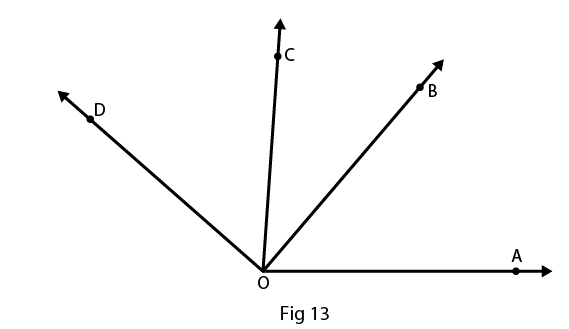Solution:
The angles that have common vertex and a common arm are known as adjacent angles
Therefore the adjacent angles in given figure are:
∠DOC and ∠BOC
∠COB and ∠BOA
2. In Fig. 14, name all the pairs of adjacent angles.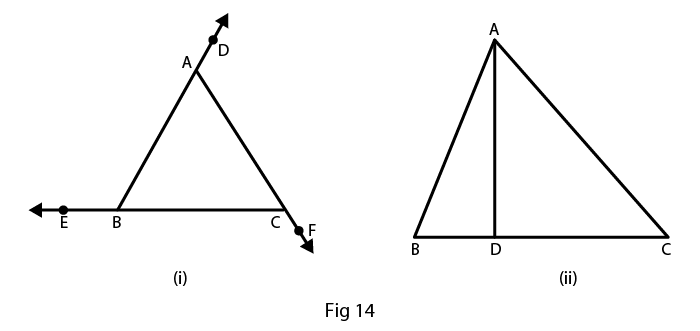Solution:
The angles that have common vertex and a common arm are known as adjacent angles.
In fig (i), the adjacent angles are
∠EBA and ∠ABC
∠ACB and ∠BCF
In fig (ii), the adjacent angles are
∠BDA and ∠CDA
3. In fig. 15, write down
(i) Each linear pair
(ii) Each pair of vertically opposite angles.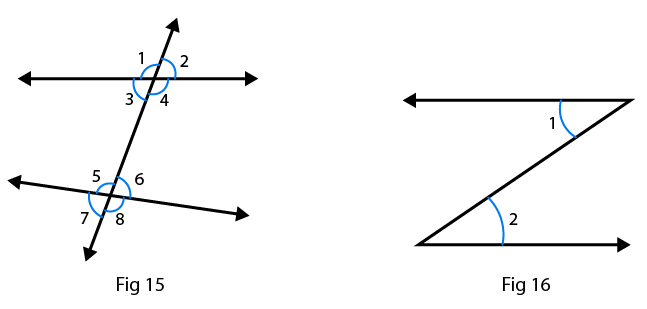Solution:
(i) The two adjacent angles are said to form a linear pair of angles if their non – common arms are two opposite rays.
∠1 and ∠3
∠1 and ∠2
∠4 and ∠3
∠4 and ∠2
∠5 and ∠6
∠5 and ∠7
∠6 and ∠8
∠7 and ∠8
(ii) The two angles formed by two intersecting lines and have no common arms are called vertically opposite angles.
∠1 and ∠4
∠2 and ∠3
∠5 and ∠8
∠6 and ∠7
4. Are the angles 1 and 2 given in Fig. 16 adjacent angles?
Solution:
No, because they don’t have common vertex.
5. Find the complement of each of the following angles:
(i) 35o
(ii) 72o
(iii) 45o
(iv) 85o
Solution:
(i) The two angles are said to be complementary angles if the sum of those angles is 90o
Complementary angle for given angle is
90o – 35o = 55o
(ii) The two angles are said to be complementary angles if the sum of those angles is 90o
Complementary angle for given angle is
900 – 72o = 18o
(iii) The two angles are said to be complementary angles if the sum of those angles is 90o
Complementary angle for given angle is
90– 45o = 45o
(iv) The two angles are said to be complementary angles if the sum of those angles is 90o
Complementary angle for given angle is
90o – 85o = 5o
6. Find the supplement of each of the following angles:
(i) 70o
(ii) 120o
(iii) 135o
(iv) 90o
Solution:
(i) The two angles are said to be supplementary angles if the sum of those angles is 180o
Therefore supplementary angle for the given question is
180o – 70o = 110o
(ii) The two angles are said to be supplementary angles if the sum of those angles is 180o
Therefore supplementary angle for the given question is
180o – 120o = 60o
(iii) The two angles are said to be supplementary angles if the sum of those angles is 180o
Therefore supplementary angle for the given question is
180o – 135o = 45o
(iv) The two angles are said to be supplementary angles if the sum of those angles is 180o
Therefore supplementary angle for the given question is
180o – 90o = 90o

7. Identify the complementary and supplementary pairs of angles from the following pairs:
(i) 25o, 65o
(ii) 120o, 60o
(iii) 63o, 27o
(iv) 100o, 80o
Solution:
(i) 25o + 65o = 90o so, this is a complementary pair of angle.
(ii) 120o + 60o = 180o so, this is a supplementary pair of angle.
(iii) 63o + 27o = 90o so, this is a complementary pair of angle.
(iv) 100o + 80o = 180o so, this is a supplementary pair of angle.
8. Can two obtuse angles be supplementary, if both of them be
(i) Obtuse?
(ii) Right?
(iii) Acute?
Solution:
(i) No, two obtuse angles cannot be supplementary
Because, the sum of two angles is greater than 90o so their sum will be greater than 180o
(ii) Yes, two right angles can be supplementary
Because, 90o + 90o = 180o
(iii) No, two acute angle cannot be supplementary
Because, the sum of two angles is less than 90o so their sum will also be less than 90o
9. Name the four pairs of supplementary angles shown in Fig.17.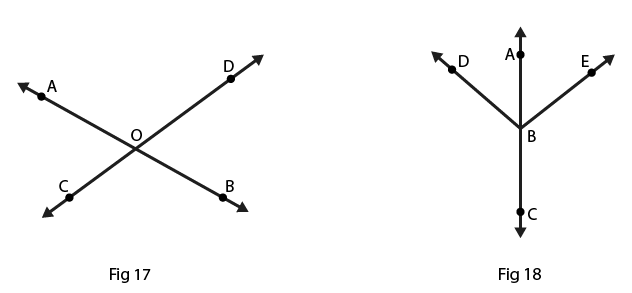Solution:
The two angles are said to be supplementary angles if the sum of those angles is 180o
The supplementary angles are
∠AOC and ∠COB
∠BOC and ∠DOB
∠BOD and ∠DOA
∠AOC and ∠DOA
10. In Fig. 18, A, B, C are collinear points and ∠DBA = ∠EBA.
(i) Name two linear pairs.
(ii) Name two pairs of supplementary angles.
Solution:
(i) Two adjacent angles are said to be form a linear pair of angles, if their non-common arms are two opposite rays.
Therefore linear pairs are
∠ABD and ∠DBC
∠ABE and ∠EBC
(ii) We know that every linear pair forms supplementary angles, these angles are
∠ABD and ∠DBC
∠ABE and ∠EBC
11. If two supplementary angles have equal measure, what is the measure of each angle?
Solution:
Let p and q be the two supplementary angles that are equal
The two angles are said to be supplementary angles if the sum of those angles is 180o
∠p = ∠q
So,
∠p + ∠q = 180o
∠p + ∠p = 180o
2∠p = 180o
∠p = 180o/2
∠p = 90o
Therefore, ∠p = ∠q = 90o
12. If the complement of an angle is 28o, then find the supplement of the angle.
Solution:
Given complement of an angle is 28o
Here, let x be the complement of the given angle 28o
Therefore, ∠x + 28o = 90o
∠x = 90o – 28o
= 62o
So, the supplement of the angle = 180o – 62o
= 118o
13. In Fig. 19, name each linear pair and each pair of vertically opposite angles: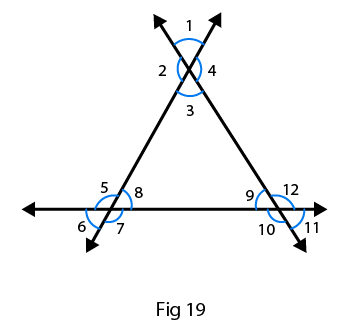Solution:
Two adjacent angles are said to be linear pair of angles, if their non-common arms are two opposite rays.
Therefore linear pairs are listed below:
∠1 and ∠2
∠2 and ∠3
∠3 and ∠4
∠1 and ∠4
∠5 and ∠6
∠6 and ∠7
∠7 and ∠8
∠8 and ∠5
∠9 and ∠10
∠10 and ∠11
∠11 and ∠12
∠12 and ∠9
The two angles are said to be vertically opposite angles if the two intersecting lines have no common arms.
Therefore supplement of the angle are listed below:
∠1 and ∠3
∠4 and ∠2
∠5 and ∠7
∠6 and ∠8
∠9 and ∠11
∠10 and ∠12
14. In Fig. 20, OE is the bisector of ∠BOD. If ∠1 = 70o, find the magnitude of ∠2, ∠3 and ∠4.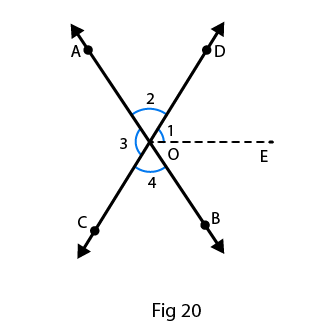Solution:
Given, ∠1 = 70o
∠3 = 2(∠1)
= 2(70o)
∠3 = 140o
∠3 = ∠4
As, OE is the angle bisector,
∠DOB = 2(∠1)
= 2(70o)
= 140o
∠DOB + ∠AOC + ∠COB +∠DOB = 360o [sum of the angle of circle = 360o]
140o + 140o + 2(∠COB) = 360o
Since, ∠COB = ∠AOD
2(∠COB) = 360o – 280o
2(∠COB) = 80o
∠COB = 80o/2
∠COB = 40o
Therefore, ∠COB = ∠AOB = 40o
The angles are, ∠1 = 70o, ∠2 = 40o, ∠3 = 140o and ∠4 = 40o
15. One of the angles forming a linear pair is a right angle. What can you say about its other angle?
Solution:
Given one of the angle of a linear pair is the right angle that is 90o
We know that linear pair angle is 180o
Therefore, the other angle is
180o – 90o = 90o
16. One of the angles forming a linear pair is an obtuse angle. What kind of angle is the other?
Solution:
Given one of the angles of a linear pair is obtuse, then the other angle should be acute, because only then their sum will be 180o.
17. One of the angles forming a linear pair is an acute angle. What kind of angle is the other?
Solution:
Given one of the Angles of a linear pair is acute, then the other angle should be obtuse, only then their sum will be 180o.
18. Can two acute angles form a linear pair?
Solution:
No, two acute angles cannot form a linear pair because their sum is always less than 180o.
19. If the supplement of an angle is 65o, then find its complement.
Solution:
Let x be the required angle
So, x + 65o = 180o
x = 180o – 65o
x = 115o
The two angles are said to be complementary angles if the sum of those angles is 90o here it is more than 90o therefore the complement of the angle cannot be determined.
20. Find the value of x in each of the following figures.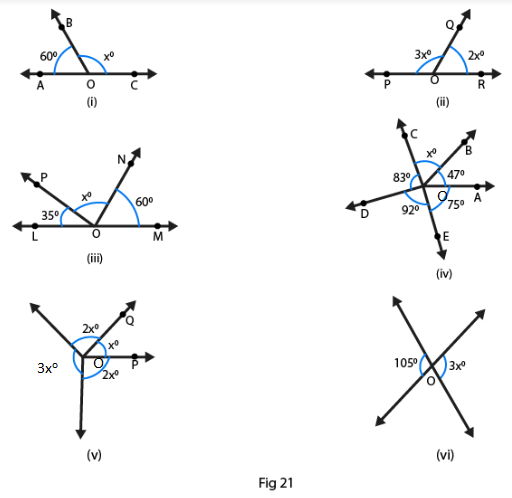Solution:
(i)  We know that ∠BOA + ∠BOC = 180o
[Linear pair: The two adjacent angles are said to form a linear pair of angles if their non–common arms are two opposite rays and sum of the angle is 180o]
60o + xo = 180o
xo = 180o – 60o
xo = 120o
(ii) We know that ∠POQ + ∠QOR = 180o
[Linear pair: The two adjacent angles are said to form a linear pair of angles if their non–common arms are two opposite rays and sum of the angle is 180o]
3xo + 2xo = 180o
5xo = 180o
xo = 180o/5
xo = 36o
(iii) We know that ∠LOP + ∠PON + ∠NOM = 180o
[Linear pair: The two adjacent angles are said to form a linear pair of angles if their non–common arms are two opposite rays and sum of the angle is 180o]
Since, 35o + xo + 60o = 180o
xo = 180o – 35o – 60o
xo = 180o – 95o
xo = 85o
(iv) We know that ∠DOC + ∠DOE + ∠EOA + ∠AOB+ ∠BOC = 360o
83o + 92o + 47o + 75o + xo = 360o
xo + 297o = 360o
xo = 360o – 297o
xo = 63o
(v) We know that ∠ROS + ∠ROQ + ∠QOP + ∠POS = 360o
3xo + 2xo + xo + 2xo = 360o
8xo = 360o
xo = 360o/8
xo = 45o
(vi)  Linear pair: The two adjacent angles are said to form a linear pair of angles if their non–common arms are two opposite rays and sum of the angle is 180o
Therefore 3xo = 105o
xo = 105o/3
xo = 45o
21. In Fig. 22, it being given that ∠1 = 65o, find all other angles.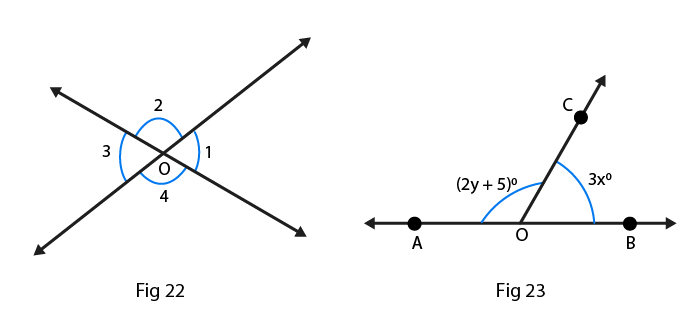Solution:
Given from the figure 22, ∠1 = ∠3 are the vertically opposite angles
Therefore, ∠3 = 65o
Here, ∠1 + ∠2 = 180° are the linear pair [The two adjacent angles are said to form a linear pair of angles if their non–common arms are two opposite rays and sum of the angle is 180o]
Therefore, ∠2 = 180o – 65o
= 115o
∠2 = ∠4 are the vertically opposite angles [from the figure]
Therefore, ∠2 = ∠4 = 115o
And ∠3 = 65o
22. In Fig. 23, OA and OB are opposite rays:
(i) If x = 25o, what is the value of y?
(ii) If y = 35o, what is the value of x?
Solution:
(i) ∠AOC + ∠BOC = 180o [The two adjacent angles are said to form a linear pair of angles if their non–common arms are two opposite rays and sum of the angle is 180o]
2y + 50 + 3x = 180o
3x + 2y = 175o
Given If x = 25o, then
3(25o) + 2y = 175o
75o + 2y = 175o
2y = 175o – 75o
2y = 100o
y = 100o/2
y = 50o
(ii) ∠AOC + ∠BOC = 180o [The two adjacent angles are said to form a linear pair of angles if their non–common arms are two opposite rays and sum of the angle is 180o]
2y + 5 + 3x = 180o
3x + 2y = 175o
Given If y = 35o, then
3x + 2(35o) = 175o
3x + 70o = 175o
3x = 1750 – 70o
3x = 105o
x = 105o/3
x = 35o
23. In Fig. 24, write all pairs of adjacent angles and all the liner pairs.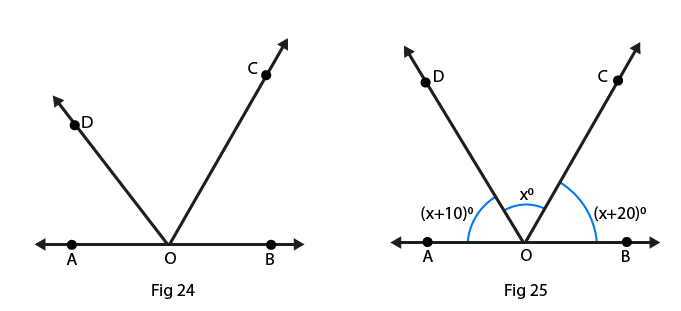Solution:
∠DOA and ∠DOC
∠BOC and ∠COD
∠AOD and ∠BOD
∠AOC and ∠BOC
Linear pairs: [The two adjacent angles are said to form a linear pair of angles if their non–common arms are two opposite rays and sum of the angle is 180o]
∠AOD and ∠BOD
∠AOC and ∠BOC
24. In Fig. 25, find ∠x. Further find ∠BOC, ∠COD and ∠AOD.
Solution:
(x + 10)o + xo + (x + 20)o = 180o[linear pair]
On rearranging we get
3xo + 30o = 180o
3xo = 180o – 30o
3xo = 150o
xo = 150o/3
xo = 50o
Also given that
∠BOC = (x + 20)o
= (50 + 20)o
= 70o
∠COD = 50o
∠AOD = (x + 10)o
= (50 + 10)o
= 60o
25. How many pairs of adjacent angles are formed when two lines intersect in a point?
Solution:
If the two lines intersect at a point, then four adjacent pairs are formed and those are linear.
26. How many pairs of adjacent angles, in all, can you name in Fig. 26?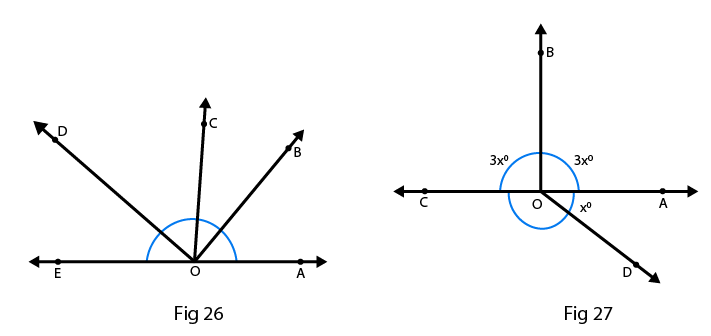Solution:
There are 10 adjacent pairs formed in the given figure, they are
∠EOD and ∠DOC
∠COD and ∠BOC
∠COB and ∠BOA
∠AOB and ∠BOD
∠BOC and ∠COE
∠COD and ∠COA
∠DOE and ∠DOB
∠EOD and ∠DOA
∠EOC and ∠AOC
∠AOB and ∠BOE
27. In Fig. 27, determine the value of x.
Solution:
From the figure we can write as ∠COB + ∠AOB = 180[linear pair]
3xo + 3xo = 180o
6xo = 180o
xo = 180o/6
xo = 30o
28. In Fig.28, AOC is a line, find x.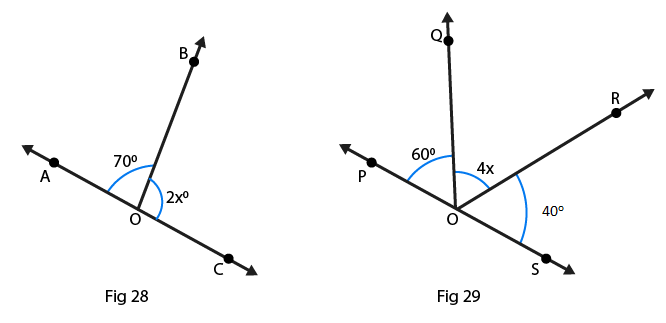Solution:
From the figure we can write as
∠AOB + ∠BOC = 180o [linear pair]
Linear pair
2x + 70o = 180o
2x = 180o – 70o
2x = 110o
x = 110o/2
x = 55o
29. In Fig. 29, POS is a line, find x.
Solution:
From the figure we can write as angles of a straight line,
∠QOP + ∠QOR + ∠ROS = 180o
60o + 4x + 40o = 180o
On rearranging we get, 100o + 4x = 180o
4x = 180o – 100o
4x = 80o
x = 80o/4
x = 20o
30. In Fig. 30, lines land l2 intersect at O, forming angles as shown in the figure. If x = 45o, find the values of y, z and u.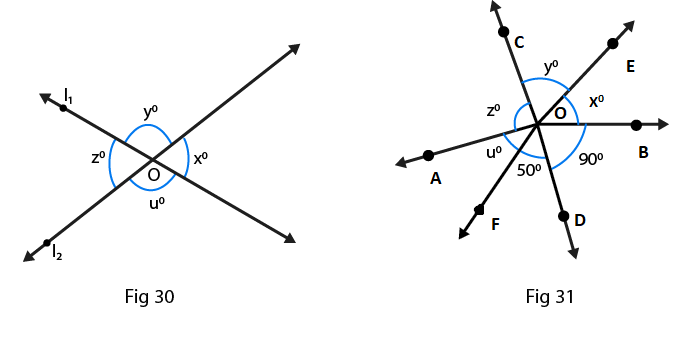Solution:
Given that, ∠x = 45o
From the figure we can write as
∠x = ∠z = 45o
Also from the figure, we have
∠y = ∠u
From the property of linear pair we can write as
∠x + ∠y + ∠z + ∠u = 360o
45o + 45o + ∠y + ∠u = 360o
90o + ∠y + ∠u = 360o
∠y + ∠u = 360o – 90o
∠y + ∠u = 270o
∠y + ∠z = 270o
2∠z = 270o
∠z = 135o
Therefore, ∠y = ∠u = 135o
So, ∠x = 45o, ∠y = 135o, ∠z = 45o and ∠u = 135o
31. In Fig. 31, three coplanar lines intersect at a point O, forming angles as shown in the figure. Find the values of x, y, z and u
Solution:
Given that, ∠x + ∠y + ∠z+ ∠u + 50o + 90o = 360o
Linear pair, ∠x + 50o + 90o = 180o
∠x + 140o = 180o
On rearranging we get
∠x = 180o – 140o
∠x = 40o
From the figure we can write as
∠x = ∠u = 40o are vertically opposite angles
∠z = 90o is a vertically opposite angle
∠y = 50o is a vertically opposite angle
Therefore, ∠x = 40o, ∠y = 50o, ∠z = 90o and ∠u = 40o
32. In Fig. 32, find the values of x, y and z.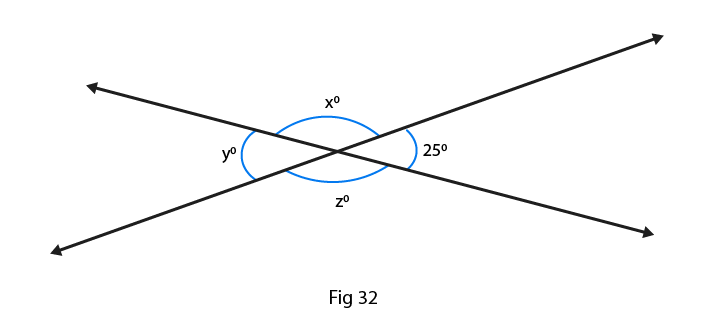Solution:
∠y = 25o vertically opposite angle
From the figure we can write as
∠x = ∠y are vertically opposite angles
∠x + ∠y + ∠z + 25o = 360o
∠x + ∠z + 25o + 25o = 360o
On rearranging we get,
∠x + ∠z + 50o = 360o
∠x + ∠z = 360o – 50o [∠x = ∠z]
2∠x = 310o
∠x = 155o
And, ∠x = ∠z = 155o
Therefore, ∠x = 155o, ∠y = 25o and ∠z = 155o

Exercise 14.2 Page No: 14.20
1. In Fig. 58, line n is a transversal to line l and m. Identify the following:
(i) Alternate and corresponding angles in Fig. 58 (i)
(ii) Angles alternate to ∠d and ∠g and angles corresponding to ∠f and ∠h in Fig. 58 (ii)
(iii) Angle alternate to ∠PQR, angle corresponding to ∠RQF and angle alternate to ∠PQE in Fig. 58 (iii)
(iv) Pairs of interior and exterior angles on the same side of the transversal in Fig. 58 (ii)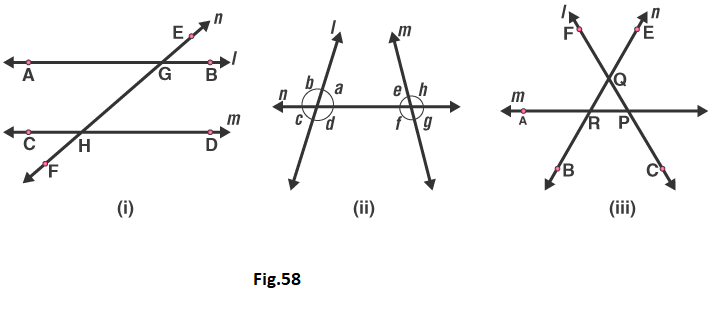Solution:
(i) A pair of angles in which one arm of both the angles is on the same side of the transversal and their other arms are directed in the same sense is called a pair of corresponding angles.
In Figure (i) Corresponding angles are
∠EGB and ∠GHD
∠HGB and ∠FHD
∠EGA and ∠GHC
∠AGH and ∠CHF
A pair of angles in which one arm of each of the angle is on opposite sides of the transversal and whose other arms include the one segment is called a pair of alternate angles.
The alternate angles are:
∠EGB and ∠CHF
∠HGB and ∠CHG
∠EGA and ∠FHD
∠AGH and ∠GHD
(ii) In Figure (ii)
The alternate angle to ∠d is ∠e.
The alternate angle to ∠g is ∠b.
The corresponding angle to ∠f is ∠c.
The corresponding angle to ∠h is ∠a.
(iii) In Figure (iii)
Angle alternate to ∠PQR is ∠QRA.
Angle corresponding to ∠RQF is ∠ARB.
Angle alternate to ∠POE is ∠ARB.
(iv) In Figure (ii)
Pair of interior angles are
∠a is ∠e.
∠d is ∠f.
Pair of exterior angles are
∠b is ∠h.
∠c is ∠g.
2. In Fig. 59, AB and CD are parallel lines intersected by a transversal PQ at L and M respectively, If ∠CMQ = 60o, find all other angles in the figure.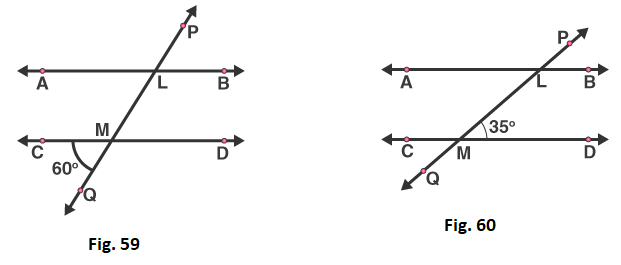Solution:
A pair of angles in which one arm of both the angles is on the same side of the transversal and their other arms are directed in the same sense is called a pair of corresponding angles.
Therefore corresponding angles are
∠ALM = ∠CMQ = 60o [given]
Vertically opposite angles are
∠LMD = ∠CMQ = 60[given]
Vertically opposite angles are
∠ALM = ∠PLB = 60o
Here, ∠CMQ + ∠QMD = 180o are the linear pair
On rearranging we get
= ∠QMD = 180o – 60o
= 120o
Corresponding angles are
∠QMD = ∠MLB = 120o
Vertically opposite angles
∠QMD = ∠CML = 120o
Vertically opposite angles
∠MLB = ∠ALP = 120o
3. In Fig. 60, AB and CD are parallel lines intersected by a transversal by a transversal PQ at L and M respectively. If ∠LMD = 35o find ∠ALM and ∠PLA.
Solution:
Given that, ∠LMD = 35o
From the figure we can write
∠LMD and ∠LMC is a linear pair
∠LMD + ∠LMC = 180[sum of angles in linear pair = 180o]
On rearranging, we get
= ∠LMC = 180o – 35o
= 145o
So, ∠LMC = ∠PLA = 145o
And, ∠LMC = ∠MLB = 145o
∠MLB and ∠ALM is a linear pair
∠MLB + ∠ALM = 180o [sum of angles in linear pair = 180o]
= ∠ALM = 180o – 145o
= ∠ALM = 350
Therefore, ∠ALM = 35o, ∠PLA = 145o.
4. The line n is transversal to line l and m in Fig. 61. Identify the angle alternate to ∠13, angle corresponding to ∠15, and angle alternate to ∠15.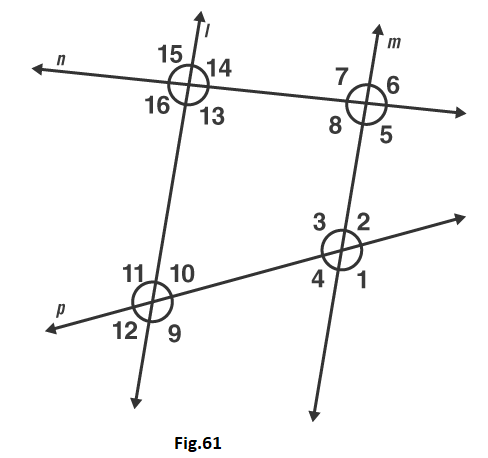Solution:
Given that, l ∥ m
From the figure the angle alternate to ∠13 is ∠7
From the figure the angle corresponding to ∠15 is ∠7 [A pair of angles in which one arm of both the angles is on the same side of the transversal and their other arms are directed in the same sense is called a pair of corresponding angles.]
Again from the figure angle alternate to ∠15 is ∠5
5. In Fig. 62, line l ∥ m and n is transversal. If ∠1 = 40°, find all the angles and check that all corresponding angles and alternate angles are equal.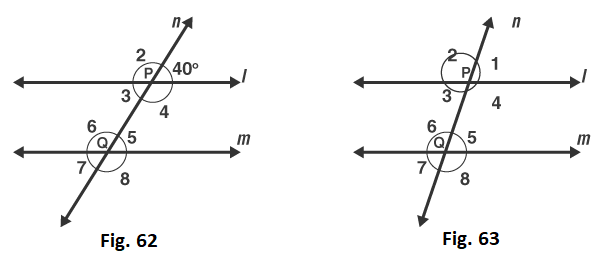Solution:
Given that, ∠1 = 40o
∠1 and ∠2 is a linear pair [from the figure]
∠1 + ∠2 = 180o
∠2 = 180o – 40o
∠2 = 140o
Again from the figure we can say that
∠2 and ∠6 is a corresponding angle pair
So, ∠6 = 140o
∠6 and ∠5 is a linear pair [from the figure]
∠6 + ∠5 = 180o
∠5 = 180o – 140o
∠5 = 40o
From the figure we can write as
∠3 and ∠5 are alternative interior angles
So, ∠5 = ∠3 = 40o
∠3 and ∠4 is a linear pair
∠3 + ∠4 = 180o
∠4 = 180o – 40o
∠4 = 140o
Now, ∠4 and ∠6 are a pair interior angles
So, ∠4 = ∠6 = 140o
∠3 and ∠7 are pair of corresponding angles
So, ∠3 = ∠7 = 40o
Therefore, ∠7 = 40o
∠4 and ∠8 are a pair corresponding angles
So, ∠4 = ∠8 = 140o
Therefore, ∠8 = 140o
Therefore, ∠1 = 40o, ∠2 = 140o, ∠3 = 40o, ∠4 = 140o, ∠5 = 40o, ∠6 = 140o, ∠7 = 40o and ∠8 = 140o
6. In Fig.63, line l ∥ m and a transversal n cuts them P and Q respectively. If ∠1 = 75°, find all other angles.
Solution:
Given that, l ∥ m and ∠1 = 75o
We know that, from the figure
∠1 + ∠2 = 180o is a linear pair
∠2 = 180o – 75o
∠2 = 105o
Here, ∠1 = ∠5 = 75o are corresponding angles
∠5 = ∠7 = 75o are vertically opposite angles.
∠2 = ∠6 = 105o are corresponding angles
∠6 = ∠8 = 105o are vertically opposite angles
∠2 = ∠4 = 105o are vertically opposite angles
So, ∠1 = 75o, ∠2 = 105o, ∠3 = 75o, ∠4 = 105o, ∠5 = 75o, ∠6 = 105o, ∠7 = 75o and ∠8 = 105o
7. In Fig. 64, AB ∥ CD and a transversal PQ cuts at L and M respectively. If ∠QMD = 100o, find all the other angles.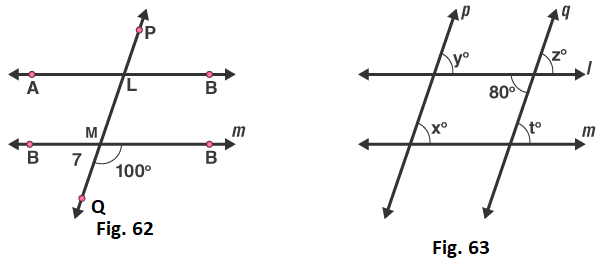Solution:
Given that, AB ∥ CD and ∠QMD = 100o
We know that, from the figure ∠QMD + ∠QMC = 180o is a linear pair,
∠QMC = 180o – ∠QMD
∠QMC = 180o – 100°
∠QMC = 80o
Corresponding angles are
∠DMQ = ∠BLM = 100o
∠CMQ = ∠ALM = 80o
Vertically Opposite angles are
∠DMQ = ∠CML = 100o
∠BLM = ∠PLA = 100o
∠CMQ = ∠DML = 80o
∠ALM = ∠PLB = 80o
8. In Fig. 65, l ∥ m and p ∥ q. Find the values of x, y, z, t.
Solution:
Given that one of the angle is 80o
∠z and 80o are vertically opposite angles
Therefore ∠z = 80o
∠z and ∠t are corresponding angles
∠z = ∠t
Therefore, ∠t = 80o
∠z and ∠y are corresponding angles
∠z = ∠y
Therefore, ∠y = 80o
∠x and ∠y are corresponding angles
∠y = ∠x
Therefore, ∠x = 80o
9. In Fig. 66, line l ∥ m, ∠1 = 120o and ∠2 = 100o, find out ∠3 and ∠4.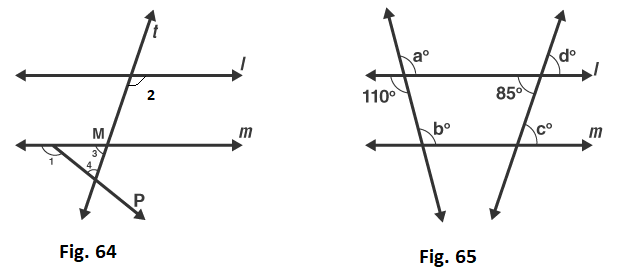Solution:
Given that, ∠1 = 120o and ∠2 = 100o
From the figure ∠1 and ∠5 is a linear pair
∠1 + ∠5 = 180o
∠5 = 180o – 120o
∠5 = 60o
Therefore, ∠5 = 60o
∠2 and ∠6 are corresponding angles
∠2 = ∠6 = 100o
Therefore, ∠6 = 100o
∠6 and ∠3 a linear pair
∠6 + ∠3 = 180o
∠3 = 180o – 100o
∠3 = 80o
Therefore, ∠3 = 80o
By, angles of sum property
∠3 + ∠5 + ∠4 = 180o
∠4 = 180o – 80o – 60o
∠4 = 40o
Therefore, ∠4 = 40o
10.  In Fig. 67, l ∥ m. Find the values of a, b, c, d. Give reasons.
Solution:
Given l ∥ m
From the figure vertically opposite angles,
∠a = 110o
Corresponding angles, ∠a = ∠b
Therefore, ∠b = 110o
Vertically opposite angle,
∠d = 85o
Corresponding angles, ∠d = ∠c
Therefore, ∠c = 85o
Hence, ∠a = 110o, ∠b = 110o, ∠c = 85o, ∠d = 85o
11. In Fig. 68, AB ∥ CD and ∠1 and ∠2 are in the ratio of 3: 2. Determine all angles from 1 to 8.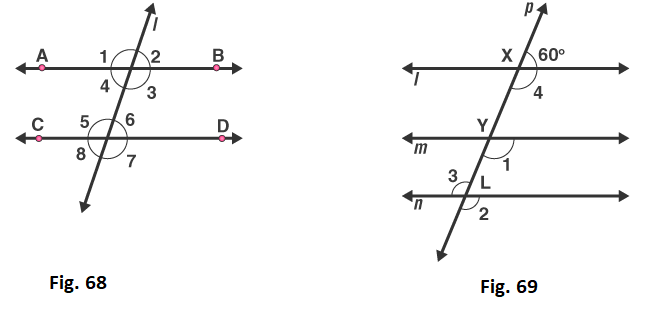Solution:
Given ∠1 and ∠2 are in the ratio 3: 2
Let us take the angles as 3x, 2x
∠1 and ∠2 are linear pair [from the figure]
3x + 2x = 180o
5x = 180o
x = 180o/5
x = 36o
Therefore, ∠1 = 3x = 3(36) = 108o
∠2 = 2x = 2(36) = 72o
∠1 and ∠5 are corresponding angles
Therefore ∠1 = ∠5
Hence, ∠5 = 108o
∠2 and ∠6 are corresponding angles
So ∠2 = ∠6
Therefore, ∠6 = 72o
∠4 and ∠6 are alternate pair of angles
∠4 = ∠6 = 72o
Therefore, ∠4 = 72o
∠3 and ∠5 are alternate pair of angles
∠3 = ∠5 = 108o
Therefore, ∠5 = 108o
∠2 and ∠8 are alternate exterior of angles
∠2 = ∠8 = 72o
Therefore, ∠8 = 72o
∠1 and ∠7 are alternate exterior of angles
∠1 = ∠7 = 108o
Therefore, ∠7 = 108o
Hence, ∠1 = 108o, ∠2 = 72o, ∠3 = 108o, ∠4 = 72o, ∠5 = 108o, ∠6 = 72o, ∠7 = 108o, ∠8 = 72o

12. In Fig. 69, l, m and n are parallel lines intersected by transversal p at X, Y and Z respectively. Find ∠1, ∠2 and ∠3.

Solution:
Given l, m and n are parallel lines intersected by transversal p at X, Y and Z
Therefore linear pair,
∠4 + 60o = 180o
∠4 = 180o – 60o
∠4 = 120o
From the figure,
∠4 and ∠1 are corresponding angles
∠4 = ∠1
Therefore, ∠1 = 120o
∠1 and ∠2 are corresponding angles
∠2 = ∠1
Therefore, ∠2 = 120o
∠2 and ∠3 are vertically opposite angles
∠2 = ∠3
Therefore, ∠3 = 1200

13. In Fig. 70, if l ∥ m ∥ n and ∠1 = 60o, find ∠2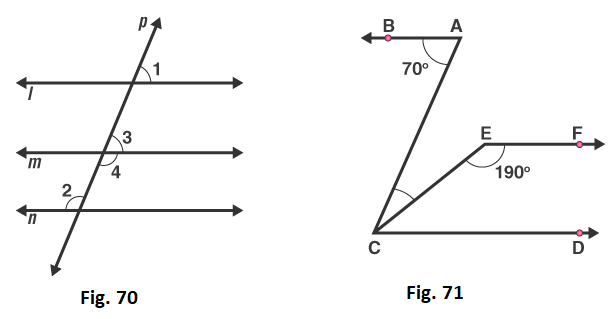Solution:
Given that l ∥ m ∥ n
From the figure Corresponding angles are
∠1 = ∠3
∠1 = 60o
Therefore, ∠3 = 60o
∠3 and ∠4 are linear pair
∠3 + ∠4 = 180o
∠4 = 180o – 60o
∠4 = 120o
∠3 and ∠4 are alternative interior angles
∠4 = ∠2
Therefore, ∠2 = 120o
14. In Fig. 71, if AB ∥ CD and CD ∥ EF, find ∠ACE
Solution:
Given that, AB ∥ CD and CD ∥ EF
Sum of the interior angles,
∠CEF + ∠ECD = 180o
130o + ∠ECD = 180o
∠ECD = 180o – 130o
∠ECD = 50o
We know that alternate angles are equal
∠BAC = ∠ACD
∠BAC = ∠ECD + ∠ACE
∠ACE = 70o – 50o
∠ACE = 20o
Therefore, ∠ACE = 20o
15. In Fig. 72, if l ∥ m, n ∥ p and ∠1 = 85o, find ∠2.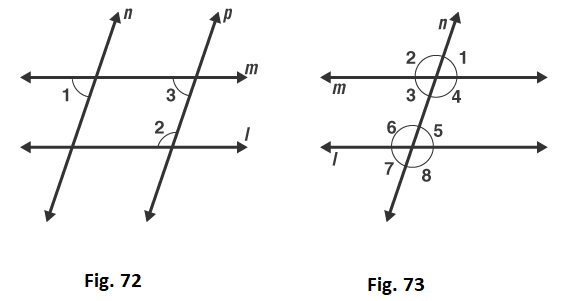Solution:
Given that, ∠1 = 85o
∠1 and ∠3 are corresponding angles
So, ∠1 = ∠3
∠3 = 85o
Sum of the interior angles is 180o
∠3 + ∠2 = 180o
∠2 = 180o – 85o
∠2 = 95o
16. In Fig. 73, a transversal n cuts two lines l and m. If ∠1 = 70o and ∠7 = 80o, is l ∥ m?
Solution:
Given ∠1 = 70o and ∠7 = 80o
We know that if the alternate exterior angles of the two lines are equal, then the lines are parallel.
Here, ∠1 and ∠7 are alternate exterior angles, but they are not equal
∠1 ≠ ∠7 ≠ 80o
17. In Fig. 74, a transversal n cuts two lines l and m such that ∠2 = 65o and ∠8 = 65o. Are the lines parallel?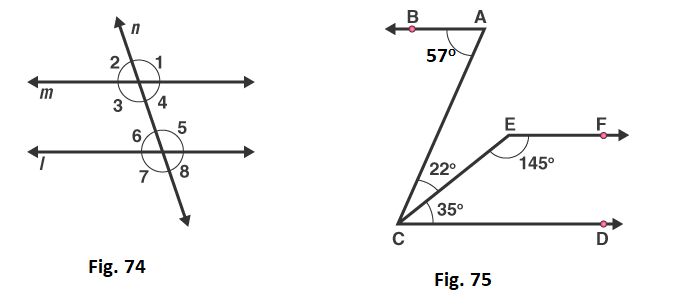Solution:
From the figure ∠2 = ∠3 are vertically opposite angels,
∠2 = ∠3 = 65o
∠8 = ∠6 = 65o
Therefore, ∠3 = ∠6
Hence, l ∥ m
18. In Fig. 75, Show that AB ∥ EF.
Solution:
We know that,
∠ACD = ∠ACE + ∠ECD
∠ACD = 35o + 22o
∠ACD = 57o = ∠BAC
Thus, lines BA and CD are intersected by the line AC such that, ∠ACD = ∠BAC
So, the alternate angles are equal
Therefore, AB ∥ CD ……1
Now,
∠ECD + ∠CEF = 35o + 45o = 180o
This, shows that sum of the angles of the interior angles on the same side of the transversal CE is 180o
So, they are supplementary angles
Therefore, EF ∥ CD …….2
From equation 1 and 2
We conclude that, AB ∥ EF
19. In Fig. 76, AB ∥ CD. Find the values of x, y, z.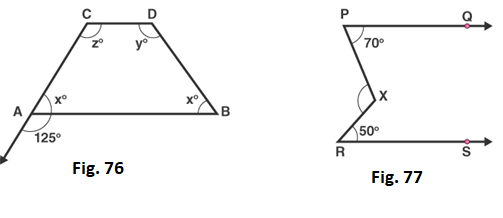Solution:
Given that AB ∥ CD
Linear pair,
∠x + 125o = 180o
∠x = 180o – 125o
∠x = 55o
Corresponding angles
∠z = 125o
∠x + ∠z = 180o
∠x + 125o = 180o
∠x = 180o – 125o
∠x = 55o
∠x + ∠y = 180o
∠y + 55o = 180o
∠y = 180o – 55o
∠y = 125o
20. In Fig. 77, find out ∠PXR, if PQ ∥ RS.
Solution:
Given PQ ∥ RS
We need to find ∠PXR
∠XRS = 50o
∠XPR = 70o
Given, that PQ ∥ RS
∠PXR = ∠XRS + ∠XPR
∠PXR = 50o + 70o
∠PXR = 120o
Therefore, ∠PXR = 120o
21. In Figure, we have
(i) ∠MLY = 2∠LMQ
(ii) ∠XLM = (2x – 10)o and ∠LMQ = (x + 30)o, find x.
(iii) ∠XLM = ∠PML, find ∠ALY
(iv) ∠ALY = (2x – 15)o, ∠LMQ = (x + 40), find x.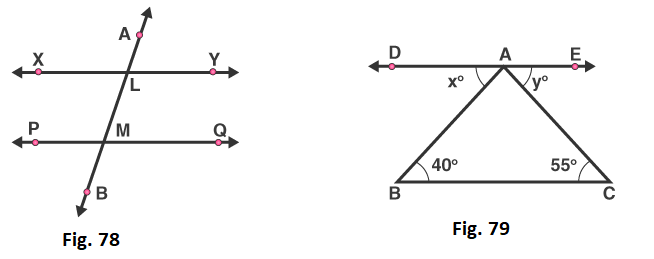Solution:
(i) ∠MLY and ∠LMQ are interior angles
∠MLY + ∠LMQ = 180o
2∠LMQ + ∠LMQ = 180o
3∠LMQ = 180o
∠LMQ = 180o/3
∠LMQ = 60o
(ii) ∠XLM = (2x – 10)o and ∠LMQ = (x + 30)o, find x.
∠XLM = (2x – 10)o and ∠LMQ = (x + 30)o
∠XLM and ∠LMQ are alternate interior angles
∠XLM = ∠LMQ
(2x – 10)o = (x + 30)o
2x – x = 30o + 10o
x = 40o
Therefore, x = 40°
(iii) ∠XLM = ∠PML, find ∠ALY
∠XLM = ∠PML
Sum of interior angles is 180 degrees
∠XLM + ∠PML = 180o
∠XLM + ∠XLM = 180o
2∠XLM = 180o
∠XLM = 180o/2
∠XLM = 90o
∠XLM and ∠ALY are vertically opposite angles
Therefore, ∠ALY = 90o
(iv) ∠ALY = (2x – 15)o, ∠LMQ = (x + 40)o, find x.
∠ALY and ∠LMQ are corresponding angles
∠ALY = ∠LMQ
(2x – 15)= (x + 40)o
2x – x = 40o + 15o
x = 55o
Therefore, x = 55o
22. In Fig. 79, DE ∥ BC. Find the values of x and y.
Solution:
We know that,
ABC, DAB are alternate interior angles
∠ABC = ∠DAB
So, x = 40o
And ACB, EAC are alternate interior angles
∠ACB = ∠EAC
So, y = 40o
23. In Fig. 80, line AC ∥ line DE and ∠ABD = 32o, Find out the angles x and y if ∠E = 122o.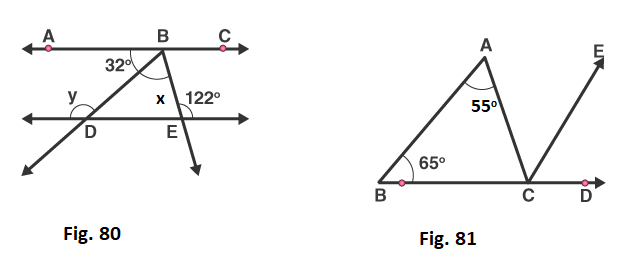Solution:
Given line AC ∥ line DE and ∠ABD = 32o
∠BDE = ∠ABD = 32o – Alternate interior angles
∠BDE + y = 180o– linear pair
32+ y = 180o
y = 180o – 32o
y = 148o
∠ABE = ∠E = 32o – Alternate interior angles
∠ABD + ∠DBE = 122o
32o + x = 122o
x = 122o – 32o
x = 90o
24. In Fig. 81, side BC of ΔABC has been produced to D and CE ∥ BA. If ∠ABC = 65o, ∠BAC = 55o, find ∠ACE, ∠ECD, ∠ACD.
Solution:
Given ∠ABC = 65o, ∠BAC = 55o
Corresponding angles,
∠ABC = ∠ECD = 55o
Alternate interior angles,
∠BAC = ∠ACE = 65o
Now, ∠ACD = ∠ACE + ∠ECD
∠ACD = 55o + 65o
= 120o
25. In Fig. 82, line CA ⊥ AB ∥ line CR and line PR ∥ line BD. Find ∠x, ∠y, ∠z.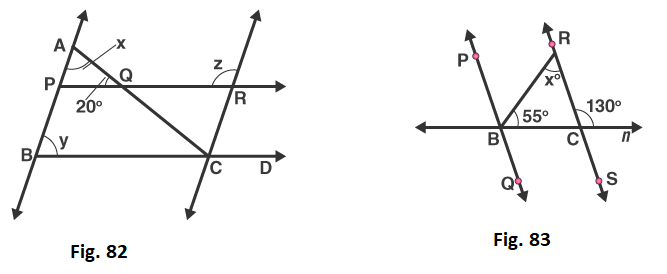Solution:
Given that, CA ⊥ AB
∠CAB = 90o
∠AQP = 20o
By, angle of sum property
In ΔAPD
∠CAB + ∠AQP + ∠APQ = 180o
∠APQ = 180o – 90o – 20o
∠APQ = 70o
y and ∠APQ are corresponding angles
y = ∠APQ = 70o
∠APQ and ∠z are interior angles
∠APQ + ∠z = 180o
∠z = 180o – 70o
∠z = 110o
26. In Fig. 83, PQ ∥ RS. Find the value of x.

Solution:
Given, linear pair,
∠RCD + ∠RCB = 180o
∠RCB = 180o – 130o
50o
In ΔABC,
∠BAC + ∠ABC + ∠BCA = 180o
By, angle sum property
∠BAC = 180o – 55o – 50o
∠BAC = 75o
27. In Fig. 84, AB ∥ CD and AE ∥ CF, ∠FCG = 90o and ∠BAC = 120o. Find the value of x, y and z.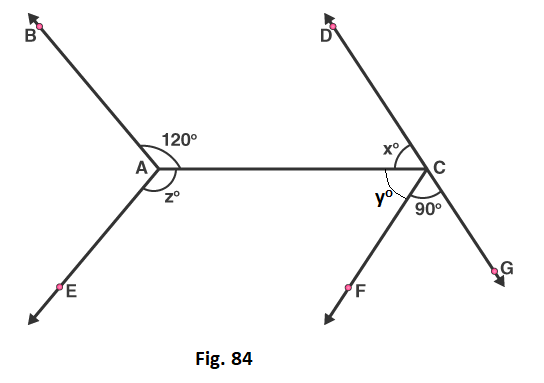Solution:
Alternate interior angle
∠BAC = ∠ACG = 120o
∠ACF + ∠FCG = 120o
So, ∠ACF = 120o – 90o
= 30o
Linear pair,
∠DCA + ∠ACG = 180o
∠x = 180o – 120o
= 60o
∠BAC + ∠BAE + ∠EAC = 360o
∠CAE = 360o – 120o – (60o + 30o)
= 150o
28. In Fig. 85, AB ∥ CD and AC ∥ BD. Find the values of x, y, z.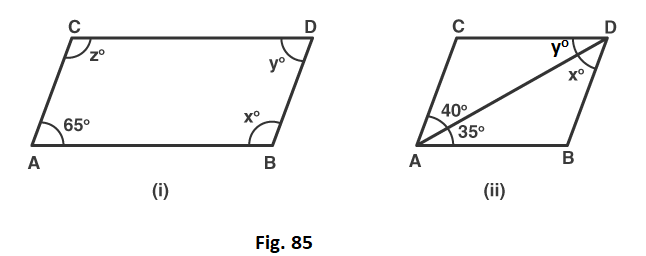Solution:
(i)  Since, AC ∥ BD and CD ∥ AB, ABCD is a parallelogram
∠ACD = 180o – 65o
= 115o
Opposite angles of parallelogram,
∠ACD = ∠DBA = 115o
(ii)  Here,
AC ∥ BD and CD ∥ AB
Alternate interior angles,
∠DCA = x = 40o
∠DAB = y = 35o
29. In Fig. 86, state which lines are parallel and why?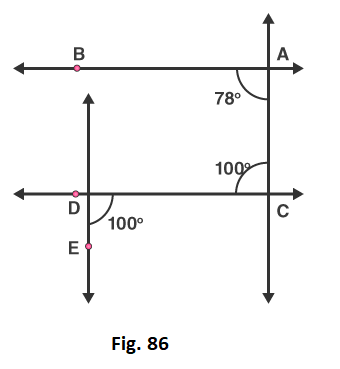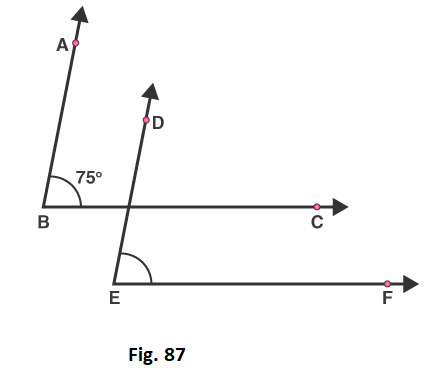Solution:
Let, F be the point of intersection of the line CD and the line passing through point E.
Here, ∠ACD and ∠CDE are alternate and equal angles.
So, ∠ACD = ∠CDE = 100o
Therefore, AC ∥ EF
30. In Fig. 87, the corresponding arms of ∠ABC and ∠DEF are parallel. If ∠ABC = 75o, find ∠DEF.
Solution:
Let, G be the point of intersection of the lines BC and DE
Since, AB ∥ DE and BC ∥ EF
The corresponding angles are,
∠ABC = ∠DGC = ∠DEF = 100o
Courtesy : CBSE